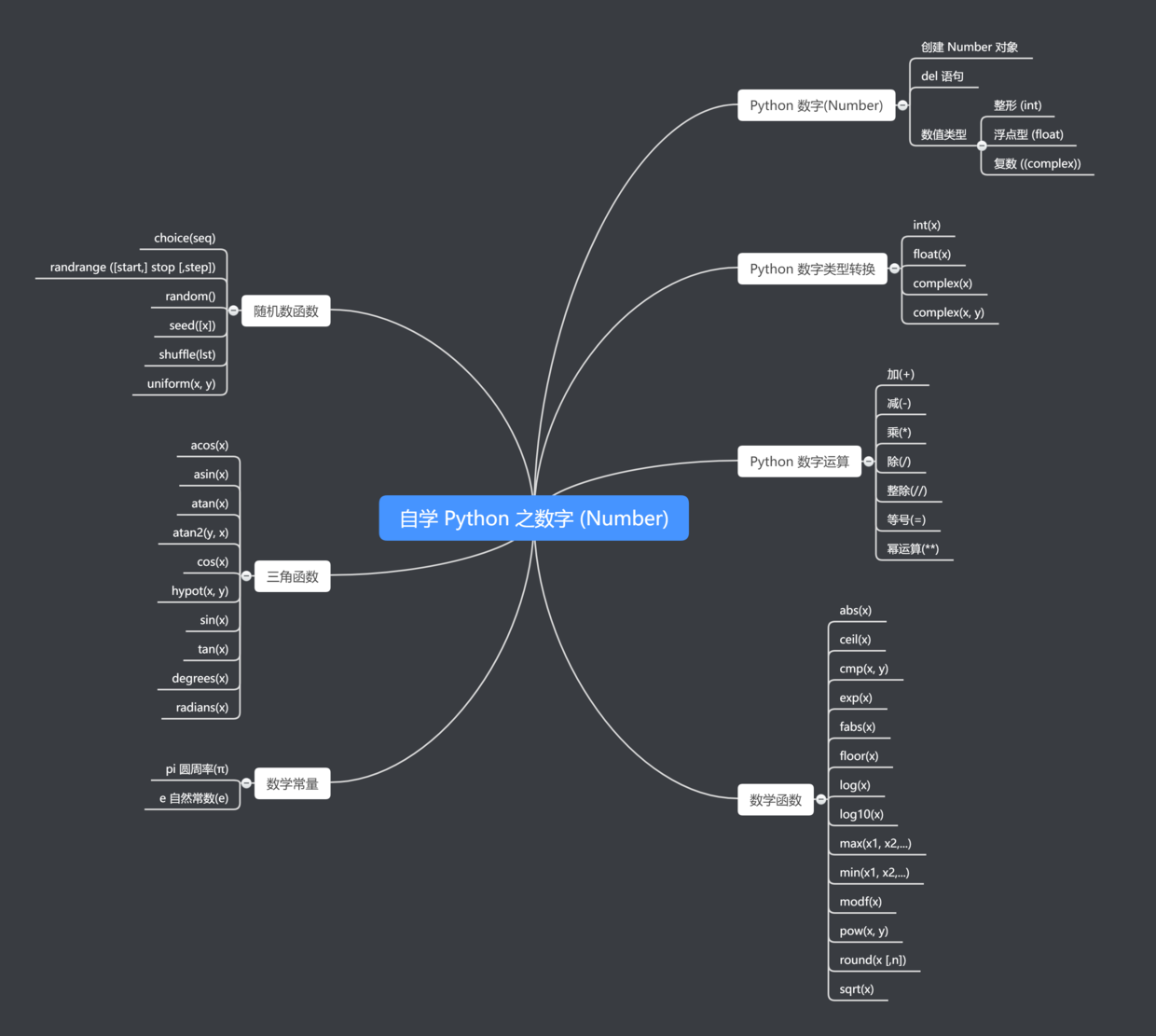## Python 实战（四）| 数字

|     |   0 浏览# 02 什么是 Python 数字(Number)

Python 数字数据类型用于存储数值。

``````var1 = 1
var2 = 10
``````

del 语句的语法是：

``````del var1[,var2[,var3[....,varN]]]]
``````

``````del var
del var_a, var_b
``````

Python 支持三种不同的数值类型：

• 整型(Int) - 通常被称为是整型或整数，是正或负整数，不带小数点。Python3 整型是没有限制大小的，可以当作 Long 类型使用，所以 Python3 没有 Python2 的 Long 类型。
• 浮点型(float) - 浮点型由整数部分与小数部分组成，浮点型也可以使用科学计数法表示（2.5e2 = 2.5 x 102 = 250）
• 复数 ( (complex)) - 复数由实数部分和虚数部分构成，可以用 a + bj ,或者 complex(a,b) 表示， 复数的实部 a 和虚部 b 都是浮点型。

``````>>> number = 0xA0F # 十六进制
>>> number
2575

>>> number=0o37 # 八进制
>>> number
31
``````
intfloatcomplex
100.03.14j
10015.2045.j
-786-21.99.322e-36j
08032.3+e18.876j
-0490-90.-.6545+0J
-0x260-32.54e1003e+26J
0x6970.2-E124.53e-7j

Python 支持复数，复数由实数部分和虚数部分构成，可以用 a + bj,或者 complex(a,b) 表示， 复数的实部 a 和虚部 b 都是浮点型。
####Python 数字类型转换

• int(x) 将x转换为一个整数。
• float(x) 将x转换到一个浮点数。
• complex(x) 将x转换到一个复数，实数部分为 x，虚数部分为 0。

complex(x, y) 将 x 和 y 转换到一个复数，实数部分为 x，虚数部分为 y。x 和 y 是数字表达式。

``````>>> a = 1.0
>>> int(a)
1
``````

# 03 Python 数字运算

Python 解释器可以作为一个简单的计算器，您可以在解释器里输入一个表达式，它将输出表达式的值。

``````>>> 2 + 2
4
>>> 50 - 5*6
20
>>> (50 - 5*6) / 4
5.0
>>> 8 / 5  # 总是返回一个浮点数
1.6
``````

**注意：**在不同的机器上浮点运算的结果可能会不一样。

``````>>> 17 / 3  # 整数除法返回浮点型
5.666666666666667
>>>
>>> 17 // 3  # 整数除法返回向下取整后的结果
5
>>> 17 % 3  # ％操作符返回除法的余数
2
>>> 5 * 3 + 2
17
``````

``````>>> 7//2
3
>>> 7.0//2
3.0
>>> 7//2.0
3.0
``````

``````>>> width = 20
>>> height = 5*9
>>> width * height
900
``````

Python 可以使用 ""** 操作来进行幂运算：

``````>>> 5 ** 2  # 5 的平方
25
>>> 2 ** 7  # 2的7次方
128
``````

``````>>> n   # 尝试访问一个未定义的变量
Traceback (most recent call last):
File "<stdin>", line 1, in <module>
NameError: name 'n' is not defined
``````

``````>>> 3 * 3.75 / 1.5
7.5
>>> 7.0 / 2
3.5
``````

``````>>> tax = 12.5 / 100
>>> price = 100.50
>>> price * tax
12.5625
>>> price + _
113.0625
>>> round(_, 2)
113.06
``````

# 04 数学函数

Python包含以下常用数学函数：

abs(x)返回数字的绝对值，如abs(-10) 返回 10
ceil(x)返回数字的上入整数，如math.ceil(4.1) 返回 5
cmp(x, y)如果 x < y 返回 -1, 如果 x == y 返回 0, 如果 x > y 返回 1。Python 3 已废弃 。使用 使用 (x>y)-(x<y) 替换。
exp(x)返回e的x次幂(e`<sup>`x`</sup>`),如math.exp(1) 返回2.718281828459045
fabs(x)返回数字的绝对值，如math.fabs(-10) 返回10.0
floor(x)返回数字的下舍整数，如math.floor(4.9)返回 4
log(x)如math.log(math.e)返回1.0,math.log(100,10)返回2.0
log10(x)返回以10为基数的x的对数，如math.log10(100)返回 2.0
max(x1, x2,...)返回给定参数的最大值，参数可以为序列。
min(x1, x2,...)返回给定参数的最小值，参数可以为序列。
modf(x)返回x的整数部分与小数部分，两部分的数值符号与x相同，整数部分以浮点型表示。
pow(x, y)x**y 运算后的值。
round(x [,n])返回浮点数x的四舍五入值，如给出n值，则代表舍入到小数点后的位数。
sqrt(x)返回数字x的平方根。

# 05 随机数函数

Python包含以下常用随机数函数：

choice(seq)从序列的元素中随机挑选一个元素，比如random.choice(range(10))，从0到9中随机挑选一个整数。
randrange ([start,] stop [,step])从指定范围内，按指定基数递增的集合中获取一个随机数，基数缺省值为1
random()随机生成下一个实数，它在[0,1)范围内。
seed([x])改变随机数生成器的种子seed。如果你不了解其原理，你不必特别去设定seed，Python会帮你选择seed。
shuffle(lst)将序列的所有元素随机排序
uniform(x, y)随机生成下一个实数，它在[x,y]范围内。

# 06 三角函数

Python包括以下三角函数：

acos(x)返回x的反余弦弧度值。
asin(x)返回x的反正弦弧度值。
atan(x)返回x的反正切弧度值。
atan2(y, x)返回给定的 X 及 Y 坐标值的反正切值。
cos(x)返回x的弧度的余弦值。
hypot(x, y)返回欧几里德范数 sqrt(xx + yy)。
sin(x)返回的x弧度的正弦值。
tan(x)返回x弧度的正切值。
degrees(x)将弧度转换为角度,如degrees(math.pi/2) ， 返回90.0

# 07 数学常量

pi数学常量 pi（圆周率，一般以π来表示）
e数学常量 e，e即自然常数（自然常数）。

# 08 后语# 09 大厂面试题 & 电子书